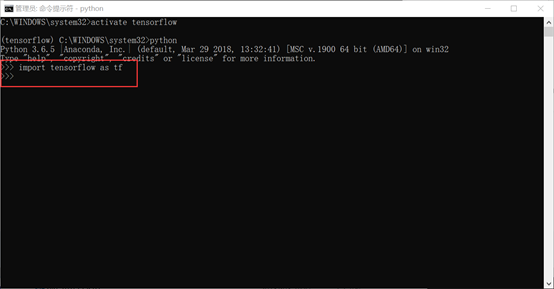# Win10 64 位Tensorflow-gpu安装（VS2017+CUDA9.2+cuDNN7.1.4+python3.6.5）

Tensorflow 入门系列的第一篇，Tensorflow-gpu的下载与安装。CSDN上能搜到很多学习资料，对初学者非常有帮助。刚安装了目前最新版本的Tensorflow，当然也碰到不少坑，这篇文章详细记录了安装过程，以供参考。

## 一 本机配置

•  Win10 64bit (版本1803)
• AMD Ryzen 5 2400G with Radeon Vega Graphics
• NVIDIA GeForce GTX 1060

## 三 安装过程

• VS2017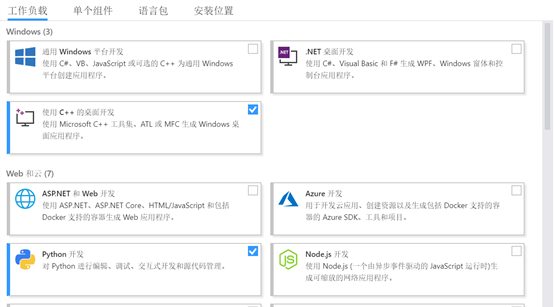• CUDA9.2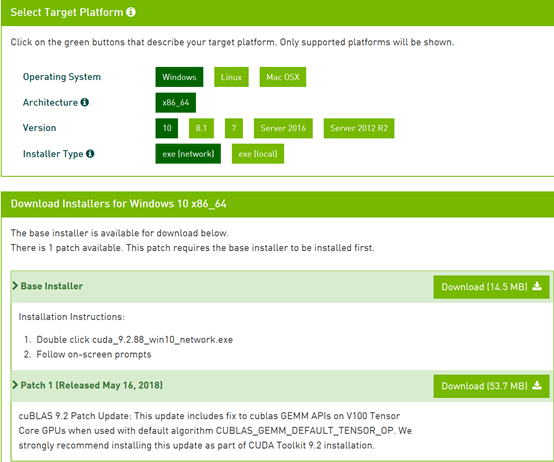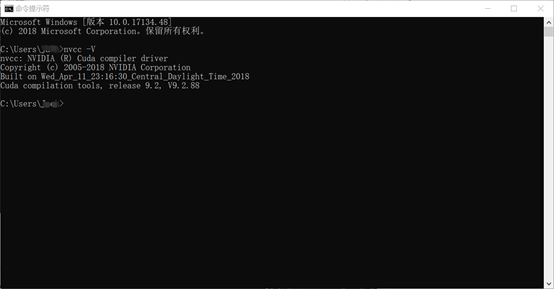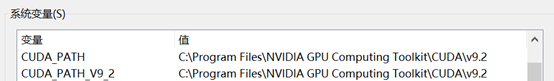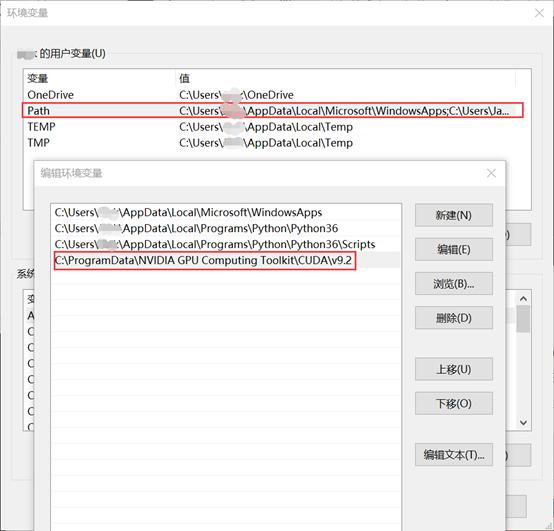•  CUDA_SDK_PATH = C:\ProgramData\NVIDIACorporation\CUDA Samples\v9.2
•  CUDA_LIB_PATH = %CUDA_PATH%\lib\x64
• CUDA_BIN_PATH = %CUDA_PATH%\bin
• CUDA_SDK_BIN_PATH = %CUDA_SDK_PATH%\bin\win64
• CUDA_SDK_LIB_PATH = %CUDA_SDK_PATH%\common\lib\x64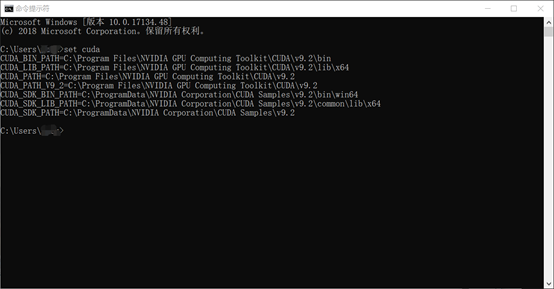• cuDNN

C:\Program Files\NVIDIA GPU Computing Toolkit\CUDA\v9.2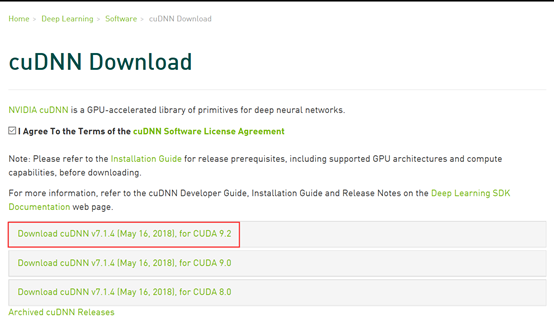• Anaconda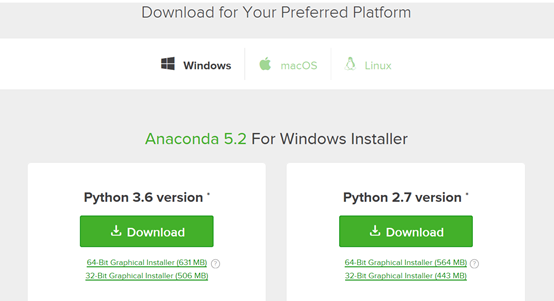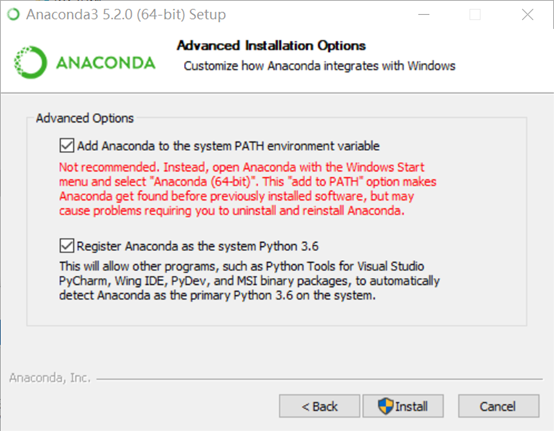• Python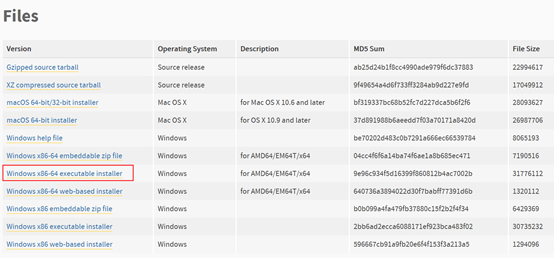conda create –n tensorflow python=3.6    下载python并安装。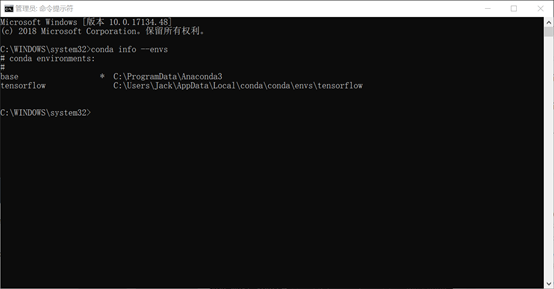• TensorFlow

https://github.com/fo40225/tensorflow-windows-wheel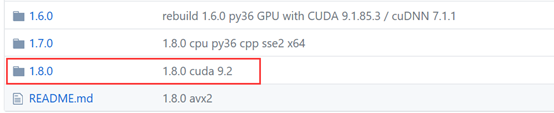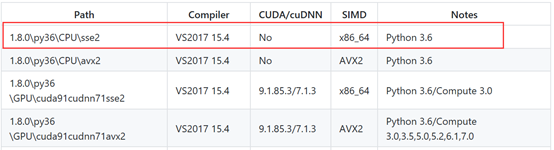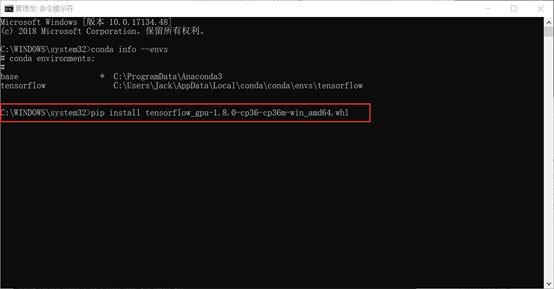• 测试TensorFlow

import tensorflow as tf